# TSA Normal Distribution Test VI

LabVIEW 2014 Advanced Signal Processing Toolkit Help

Edition Date: June 2014

Part Number: 372656C-01

»View Product InfoDownload Help (Windows Only)

Owning Palette: Statistical Analysis VIs

Requires: Advanced Signal Processing Toolkit

Determines if a univariate time series is normally distributed by comparing the discrete histogram of the time series with the assumed normal distribution histogram that this VI generates according to the mean and variance values of the time series. Wire data to the Xt input to determine the polymorphic instance to use or manually select the instance.

Examples

Use the pull-down menu to select an instance of this VI.

 Select an instance TSA Normal Distribution Test (Waveform)TSA Normal Distribution Test (Array)

## TSA Normal Distribution Test (Waveform)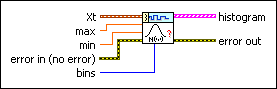Xt specifies the univariate time series.max specifies the maximum value to include in the histogram.min specifies the minimum value to include in the histogram.error in describes error conditions that occur before this node runs. This input provides standard error in functionality.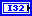bins specifies the number of bins in the histogram. The default is 0.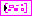histogram returns the histogram data of the univariate time series and assumed histogram data of normal distribution.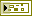error out contains error information. This output provides standard error out functionality.

## TSA Normal Distribution Test (Array)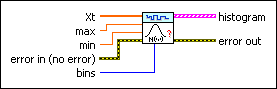Xt specifies the univariate time series.max specifies the maximum value to include in the histogram.min specifies the minimum value to include in the histogram.error in describes error conditions that occur before this node runs. This input provides standard error in functionality.bins specifies the number of bins in the histogram. The default is 0.histogram returns the histogram data of the univariate time series and assumed histogram data of normal distribution.error out contains error information. This output provides standard error out functionality.

## Examples

Refer to the following VIs for examples of using the TSA Normal Distribution Test VI:

• Random Series Generate VI: labview\examples\Time Series Analysis\TSAGettingStarted
• Series Statistical Analysis VI: labview\examples\Time Series Analysis\TSAGettingStarted

Not Helpful